# 萬字長文帶你漫遊資料結構世界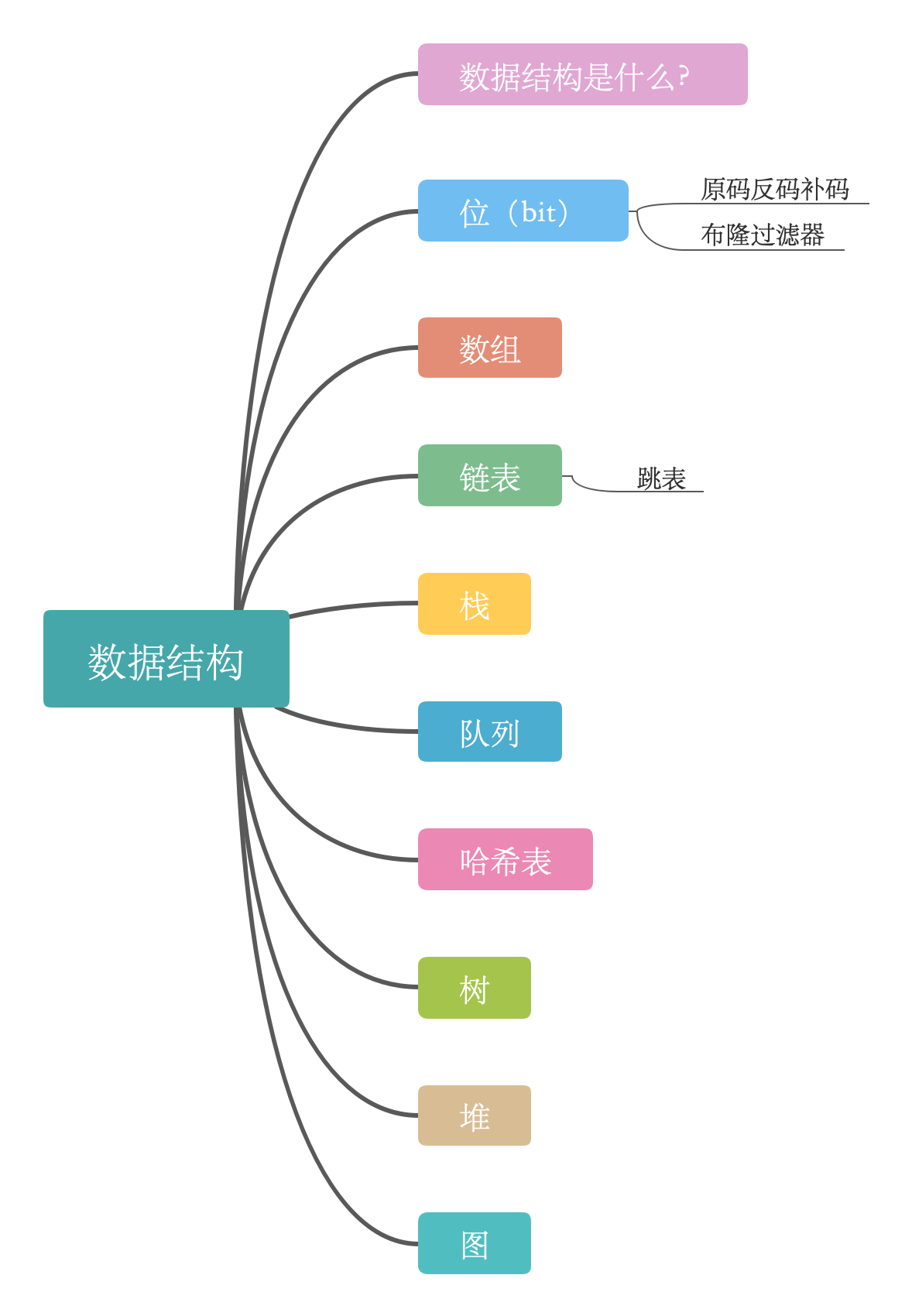# 資料結構是什麼？

• 集合：只有同屬於一個集合的關係，沒有其他關係
• 線性結構：結構中的資料元素之間存在一個對一個的關係
• 樹形結構：結構中的資料元素之間存在一個對多個的關係
• 圖狀結構或者網狀結構：圖狀結構或者網狀結構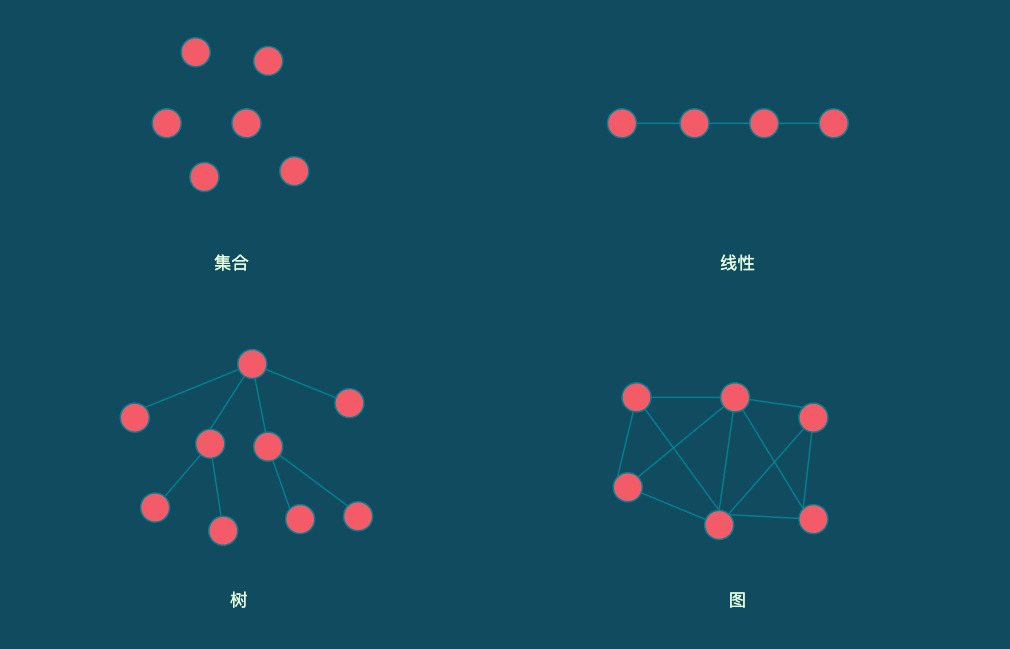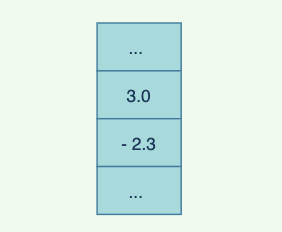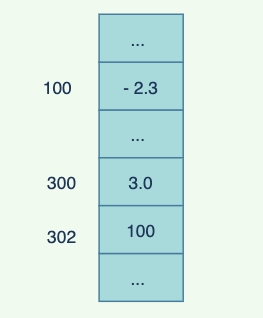## 位（bit）

• 原碼：用第一位表示符號，其餘位表示值
• 反碼：正數的補碼反碼是其本身，負數的反碼是符號位保持不變，其餘位取反。
• 補碼：正數的補碼是其本身，負數的補碼是在其反碼的基礎上 + 1

### 為什麼有了原碼還要反碼和補碼？

``````        00100011		35
+      11011101	   -35
-------------------------
00000000       0
``````
``````        00100011		35
+ 	    11011011	   -37
-------------------------
11111110       -2
``````

• `~`：按位取反
• `&`：按為與運算
• `|`：按位或運算
• `^`：按位異或
• `<<`: 帶符號左移，比如`35(00100011)`,左移一位為 `70(01000110)`,`-35(11011101)`左移一位為`-70(10111010)`
• `>>`：帶符號右移，比如`35(00100011)`,右移一位為 `17(00010001)`,`-35(11011101)`左移一位為`-18(11101110)`
• `<<<`:無符號左移，比如`35(00100011)`,左移一位為`70(01000110)`
• `>>>`:無符號右移，比如`-35(11011101)`,右移一位為`110(01101110)`
• `x ^= y; y ^= x; x ^= y;`:交換
• `s &= ~(1 << k)`:第`k`位置0

### 布隆過濾器是什麼呢？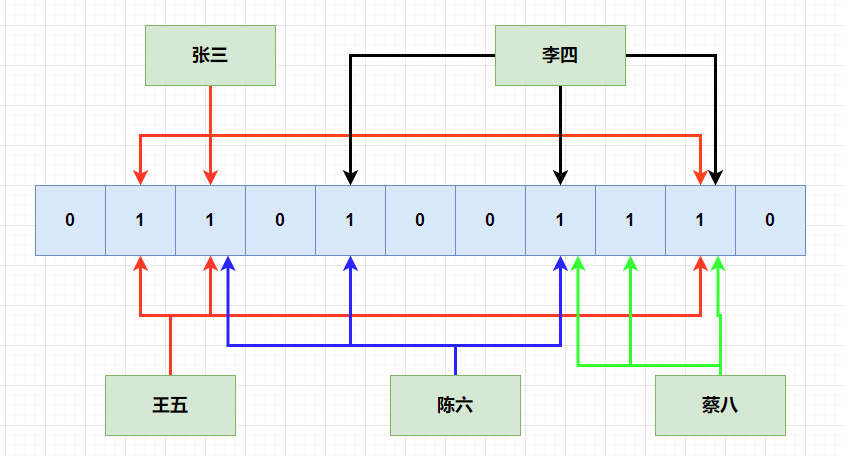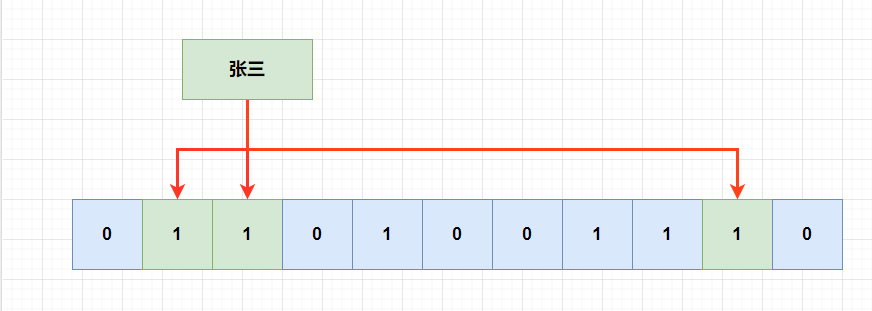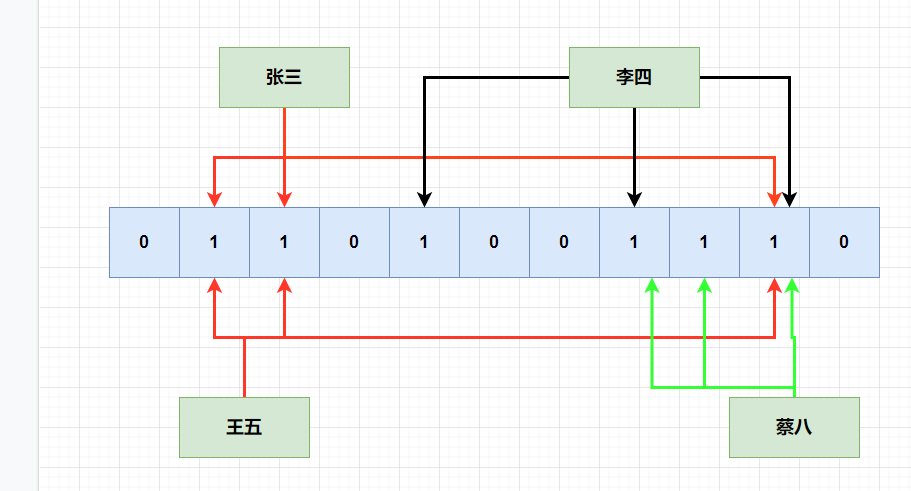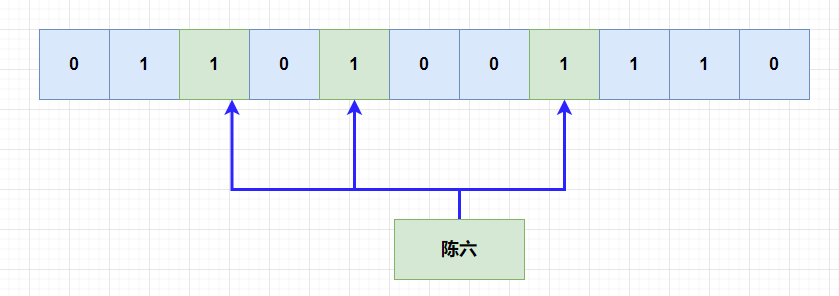## 陣列

• 存在唯一的第一個的資料元素
• 存在唯一被稱為最後一個的資料元素
• 除了第一個以外，集合中每一個元素均有一個前驅
• 除了最後一個元素之外，集合中的每一個數據元素都有一個後繼元素

• 陣列：查詢 / 更新快，查詢/刪除慢
• 連結串列
• 佇列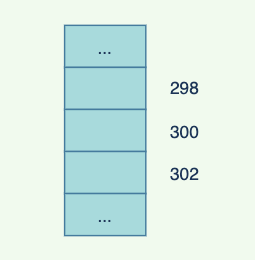`Java`中表示為：

``````int[] nums = new int;
int[] nums = {1,2,3,4,5};

Object[] Objects = new Object;
``````

`C++` 中表示為：

``````int nums;
``````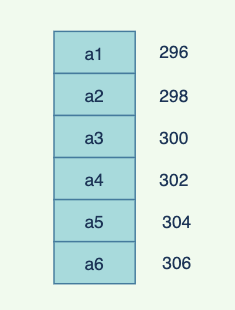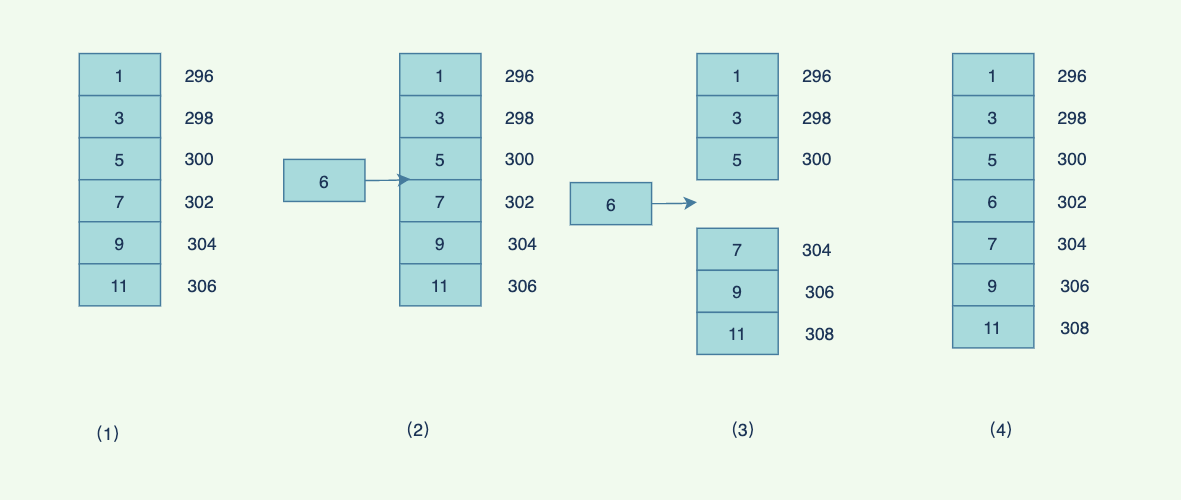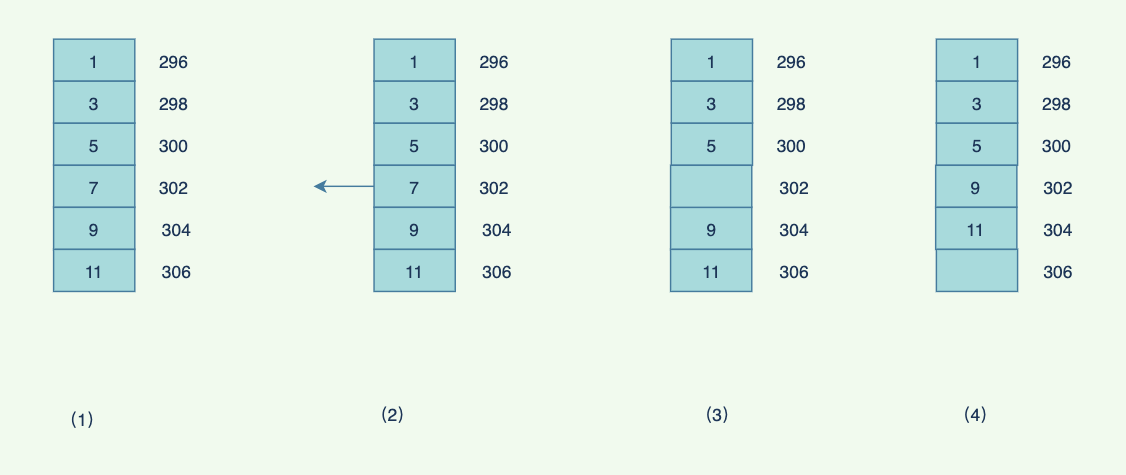Java程式碼實現陣列的增刪改查：

``````package datastruction;

import java.util.Arrays;

public class MyArray {
private int[] data;

private int elementCount;

private int length;

public MyArray(int max) {
length = max;
data = new int[max];
elementCount = 0;
}

if (elementCount == length) {
length = 2 * length;
data = Arrays.copyOf(data, length);
}
data[elementCount] = value;
elementCount++;
}

public int find(int searchKey) {
int i;
for (i = 0; i < elementCount; i++) {
if (data[i] == searchKey)
break;
}
if (i == elementCount) {
return -1;
}
return i;
}

public boolean delete(int value) {
int i = find(value);
if (i == -1) {
return false;
}
for (int j = i; j < elementCount - 1; j++) {
data[j] = data[j + 1];
}
elementCount--;
return true;
}

public boolean update(int oldValue, int newValue) {
int i = find(oldValue);
if (i == -1) {
return false;
}
data[i] = newValue;
return true;
}
}

// 測試類
public class Test {
public static void main(String[] args) {
MyArray myArray = new MyArray(2);
myArray.delete(2);
System.out.println(myArray);
}
}
``````

## 連結串列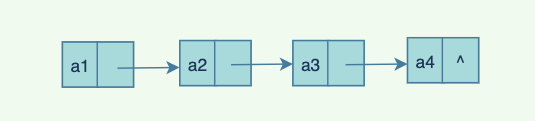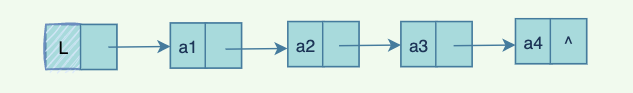• 單向連結串列：連結串列中的每一個結點，都有且只有一個指標指向下一個結點，並且最後一個節點指向空。
• 雙向連結串列：每個節點都有兩個指標（為方便，我們稱之為前指標後指標），分別指向上一個節點和下一個節點，第一個節點的前指標指向`NULL`，最後一個節點的後指標指向`NULL`
• 迴圈連結串列：每一個節點的指標指向下一個節點，並且最後一個節點的指標指向第一個節點（雖然是迴圈連結串列，但是必要的時候還需要標識頭結點或者尾節點，避免死迴圈）
• 複雜連結串列：每一個連結串列有一個後指標，指向下一個節點，同時有一個隨機指標，指向任意一個結點。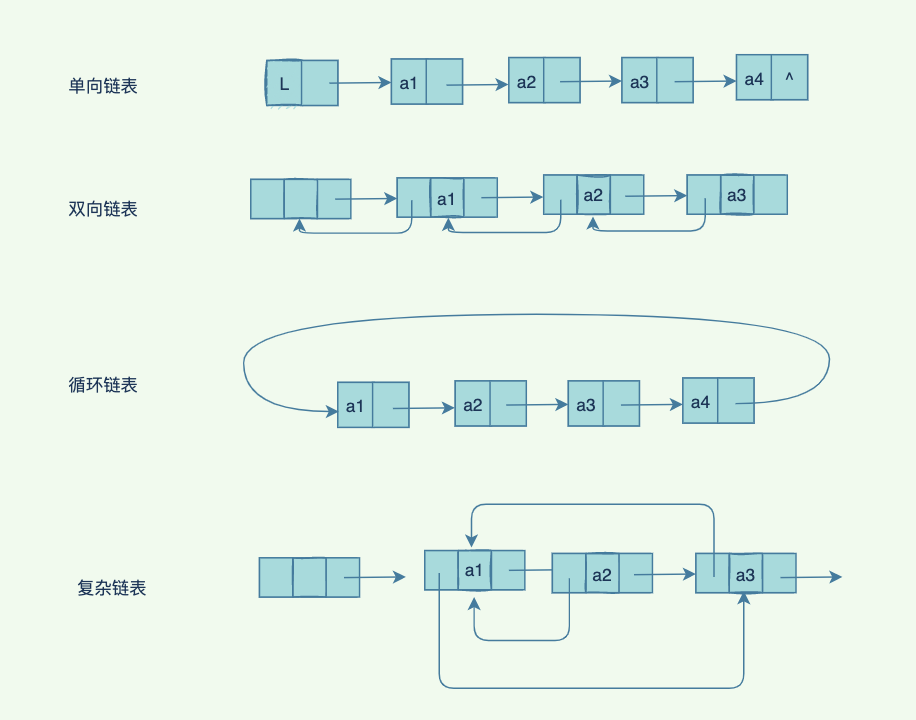• 查詢：`O(n)`,需要遍歷連結串列
• 插入：`O(1)`，修改前後指標即可
• 刪除：`O(1)`，同樣是修改前後指標即可
• 修改：不需要查詢則為`O(1)`，需要查詢則為`O(n)`

``````// 結點
typedef struct LNode{
// 資料
ElemType data;
// 下一個節點的指標
struct LNode *next;

// 連結串列
typedef struct{
// 頭結點，尾節點
// 長度
int len;
``````

`Java` 程式碼表示：

``````    public class ListNode {
int val;
ListNode next = null;

ListNode(int val) {
this.val = val;
}
}
``````

``````class ListNode<T> {
T val;
ListNode next = null;

ListNode(T val) {
this.val = val;
}
}

public class MyList<T> {
private ListNode<T> tail;
private int size;

public MyList() {
this.tail = null;
this.size = 0;
}

}

public void add(int index, T element) {
if (index < 0 || index > size) {
throw new IndexOutOfBoundsException("超出連結串列長度範圍");
}
ListNode current = new ListNode(element);
if (index == 0) {
tail = current;
} else {
}
} else if (index == size) {
tail.next = current;
tail = current;
} else {
ListNode preNode = get(index - 1);
current.next = preNode.next;
preNode.next = current;
}
size++;
}

public ListNode get(int index) {
if (index < 0 || index >= size) {
throw new IndexOutOfBoundsException("超出連結串列長度");
}
for (int i = 0; i < index; i++) {
temp = temp.next;
}
return temp;
}

public ListNode delete(int index) {
if (index < 0 || index >= size) {
throw new IndexOutOfBoundsException("超出連結串列節點範圍");
}
ListNode node = null;
if (index == 0) {
} else if (index == size - 1) {
ListNode preNode = get(index - 1);
node = tail;
preNode.next = null;
tail = preNode;
} else {
ListNode pre = get(index - 1);
pre.next = pre.next.next;
node = pre.next;
}
size--;
return node;
}

public void update(int index, T element) {
if (index < 0 || index >= size) {
throw new IndexOutOfBoundsException("超出連結串列節點範圍");
}
ListNode node = get(index);
node.val = element;
}

public void display() {
while (temp != null) {
System.out.print(temp.val + " -> ");
temp = temp.next;
}
System.out.println("");
}
}

``````

``````public class Test {
public static void main(String[] args) {
MyList myList = new MyList();
// 1->2
myList.display();

// 1
System.out.println(myList.get(0).val);

myList.update(1,3);
// 1->3
myList.display();

// 1->3->4
myList.display();

myList.delete(1);
// 1->4
myList.display();
}
}
``````

``````1 -> 2 ->
1
1 -> 3 ->
1 -> 3 -> 4 ->
1 -> 4 ->
``````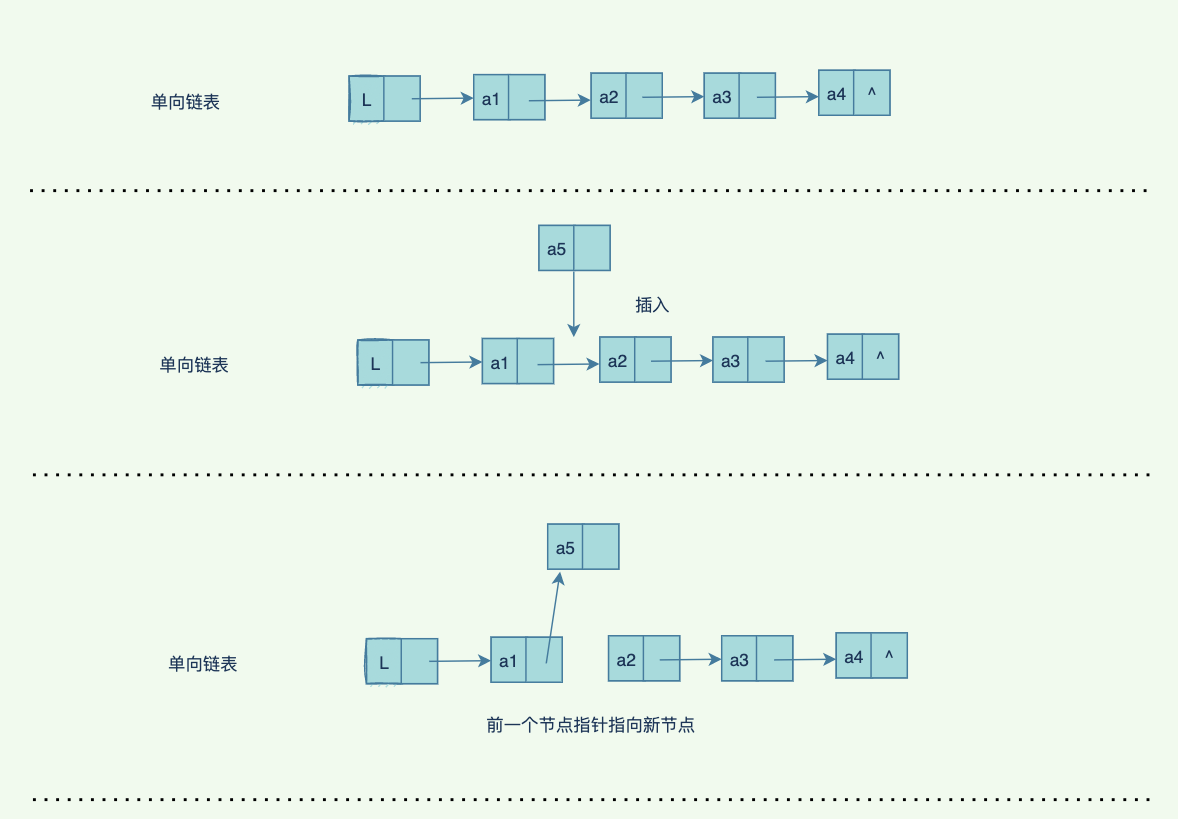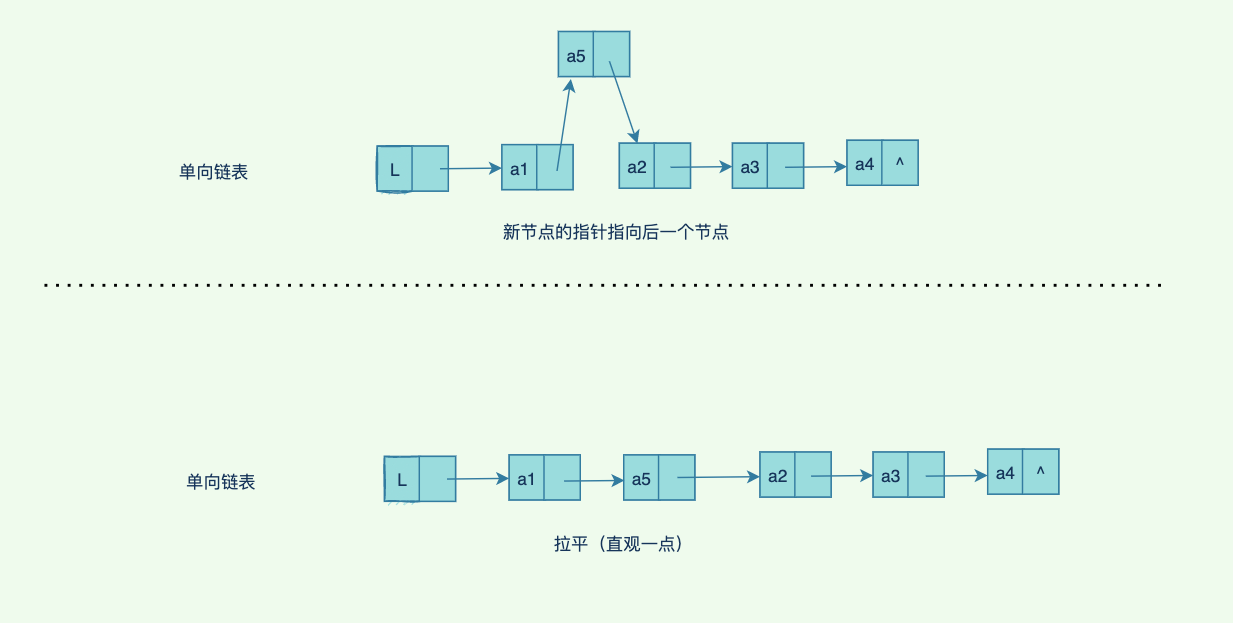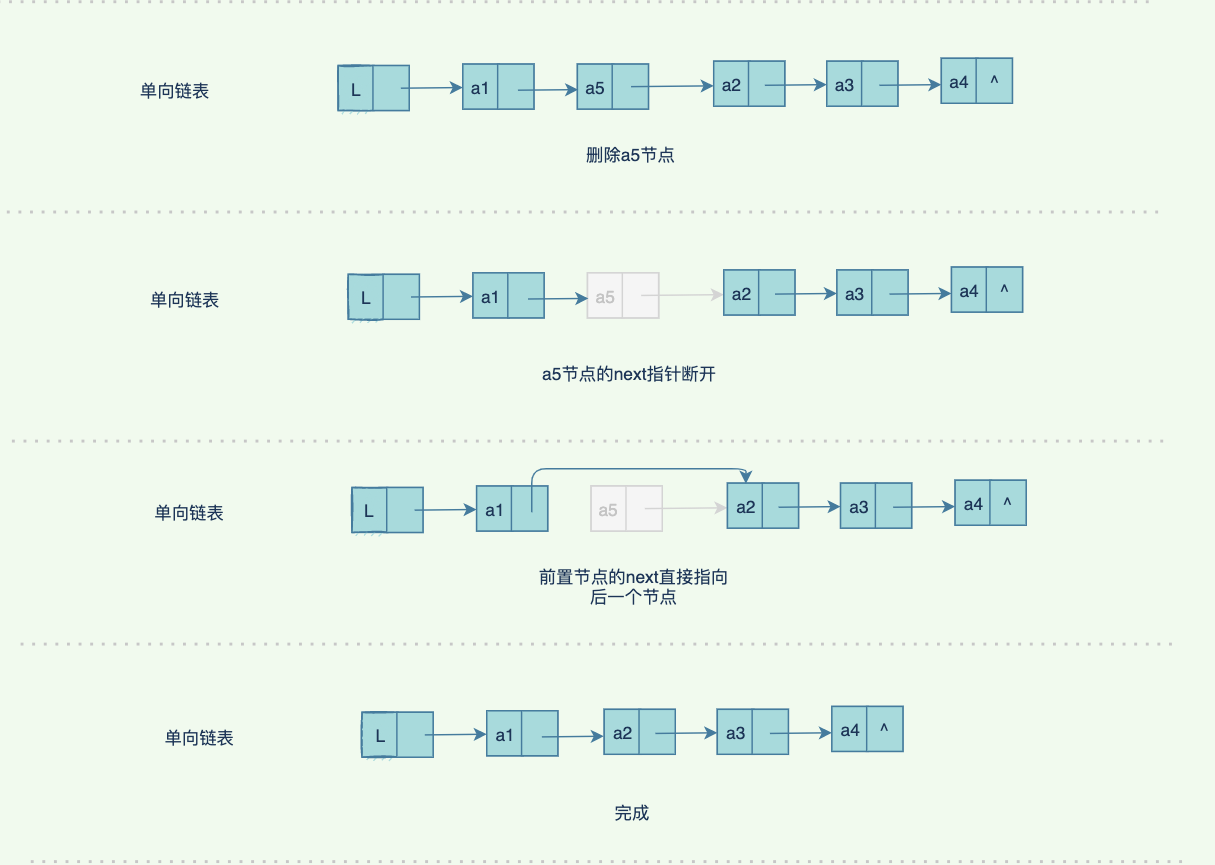### 跳錶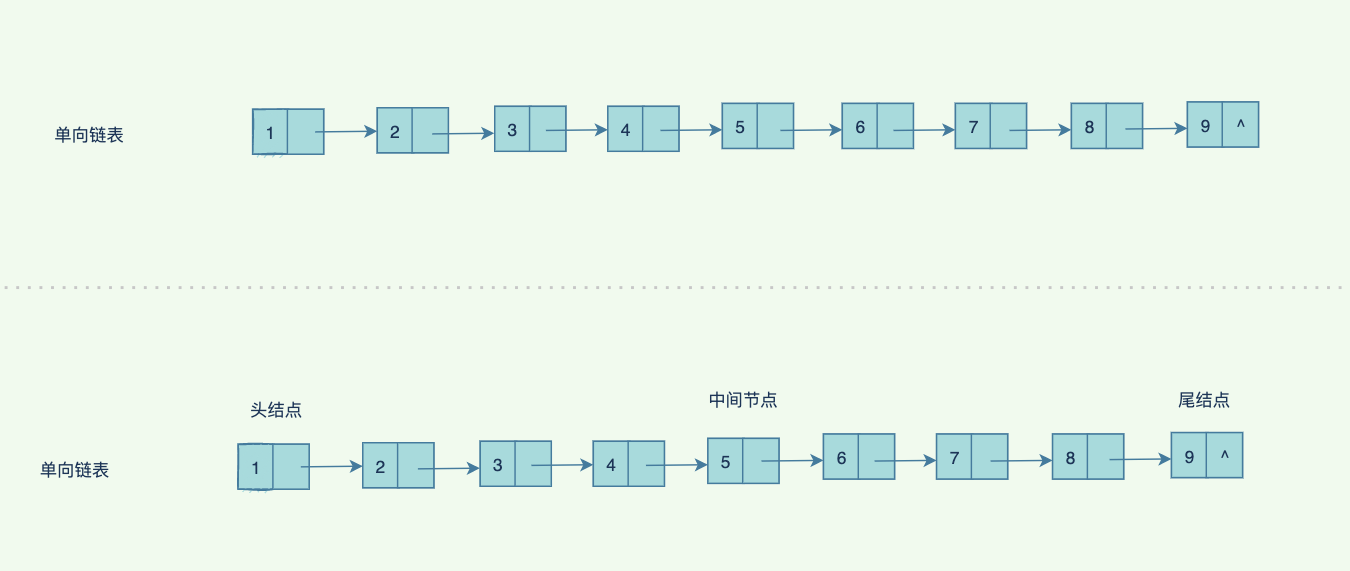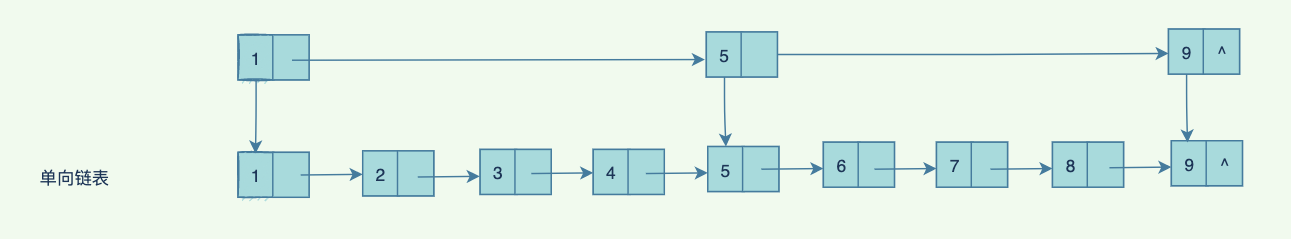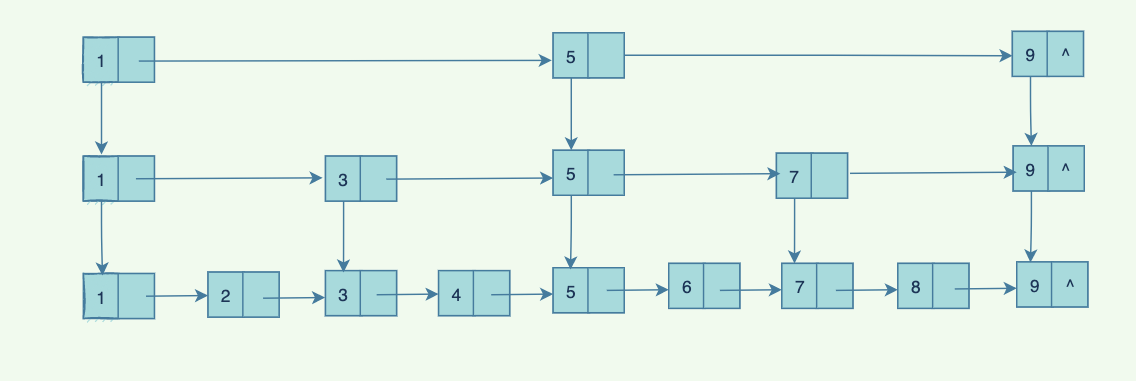`redis` 中使用了跳錶來實現`zset`,`redis`中使用一個隨機演算法來計算層級，計算出每個節點到底多少層索引，雖然不能絕對保證比較平衡，但是基本保證了效率，實現起來比那些平衡樹，紅黑樹的演算法簡單一點。

## 棧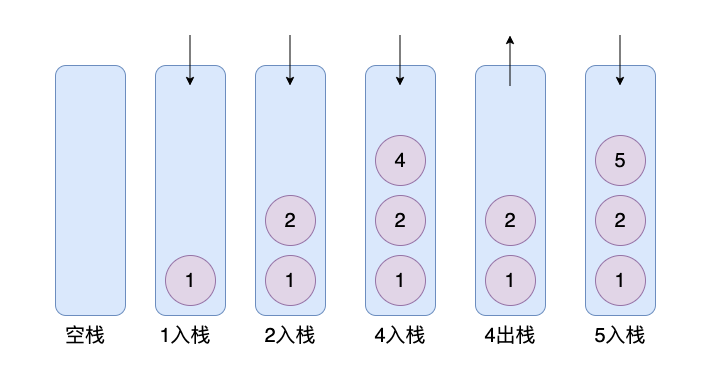``````import java.util.Arrays;

public class MyStack<T> {
private T[] data;
private int length = 2;
private int maxIndex;

public MyStack() {
data = (T[]) new Object[length];
maxIndex = -1;
}

public void push(T element) {
if (isFull()) {
length = 2 * length;
data = Arrays.copyOf(data, length);
}
data[maxIndex + 1] = element;
maxIndex++;
}

public T pop() {
if (isEmpty()) {
throw new IndexOutOfBoundsException("棧內沒有資料");
} else {
T[] newdata = (T[]) new Object[data.length - 1];
for (int i = 0; i < data.length - 1; i++) {
newdata[i] = data[i];
}
T element = data[maxIndex];
maxIndex--;
data = newdata;
return element;
}
}

private boolean isFull() {
return data.length - 1 == maxIndex;
}

public boolean isEmpty() {
return maxIndex == -1;
}

public void display() {
for (int i = 0; i < data.length; i++) {
System.out.print(data[i]+" ");
}
System.out.println("");
}
}

``````

``````public class MyStackTest {
public static void main(String[] args) {
MyStack<Integer> myStack = new MyStack<>();
myStack.push(1);
myStack.push(2);
myStack.push(3);
myStack.push(4);
myStack.display();

System.out.println(myStack.pop());

myStack.display();

}
}
``````

``````1 2 3 4
4
1 2 3
``````

## 佇列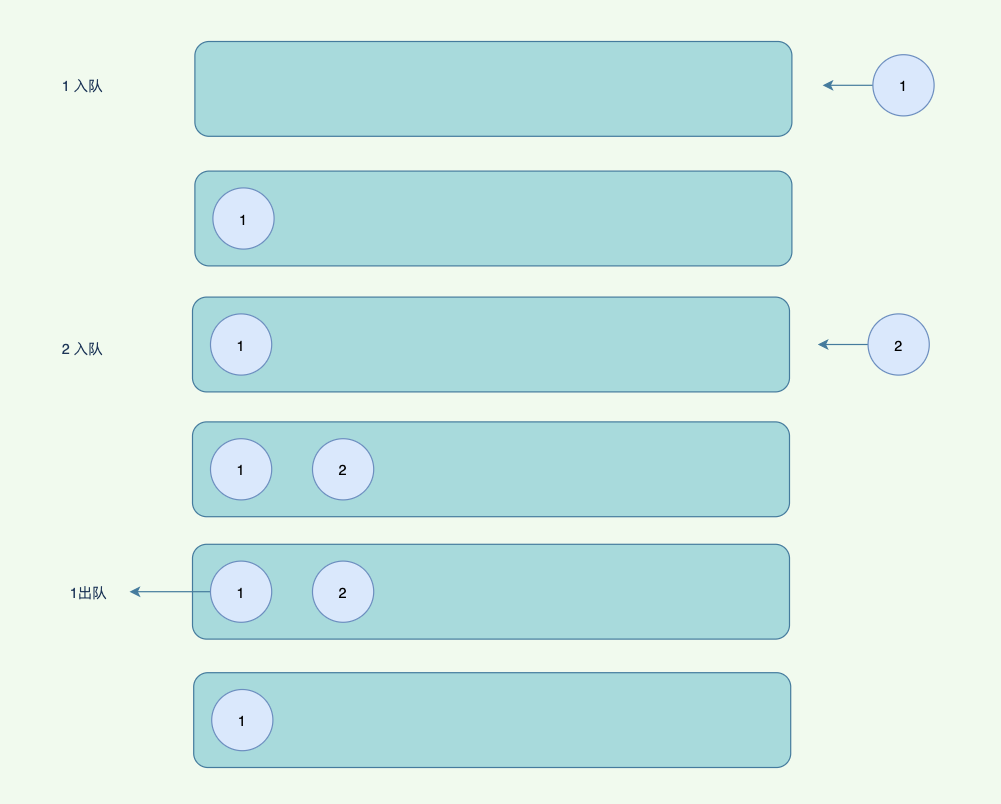``````class Node<T> {
public T data;
public Node next;

public Node(T data) {
this.data = data;
}
}

public class MyQueue<T> {
private Node<T>  rear;
private int size;

public MyQueue() {
size = 0;
}

public void pushBack(T element) {
Node newNode = new Node(element);
if (isEmpty()) {
} else {
rear.next = newNode;
}
rear = newNode;
size++;
}

public boolean isEmpty() {
}

public T popFront() {
if (isEmpty()) {
throw new NullPointerException("佇列沒有資料");
} else {
size--;
return node.data;
}
}

public void dispaly() {
while (temp != null) {
System.out.print(temp.data +" -> ");
temp = temp.next;
}
System.out.println("");
}
}
``````

``````public class MyStackTest {
public static void main(String[] args) {
MyStack<Integer> myStack = new MyStack<>();
myStack.push(1);
myStack.push(2);
myStack.push(3);
myStack.push(4);
myStack.display();

System.out.println(myStack.pop());

myStack.display();

}
}
``````

``````1 -> 2 -> 3 ->
1
2 -> 3 ->
2
3 ->
``````

• 單向佇列：也就是我們說的普通佇列，先進先出。

• 雙向佇列：可以從不同方向進出佇列

• 優先佇列：內部是自動排序的，按照一定順序出佇列

• 阻塞佇列：從佇列取出元素的時候，佇列沒有元素則會阻塞，同樣如果佇列滿了，往佇列裡面放入元素也會被阻塞。

• 迴圈佇列：可以理解為一個迴圈連結串列，但是一般需要標識出頭尾節點，防止死迴圈，尾節點的`next`指向頭結點。

## 雜湊表

`hash`表定義如下：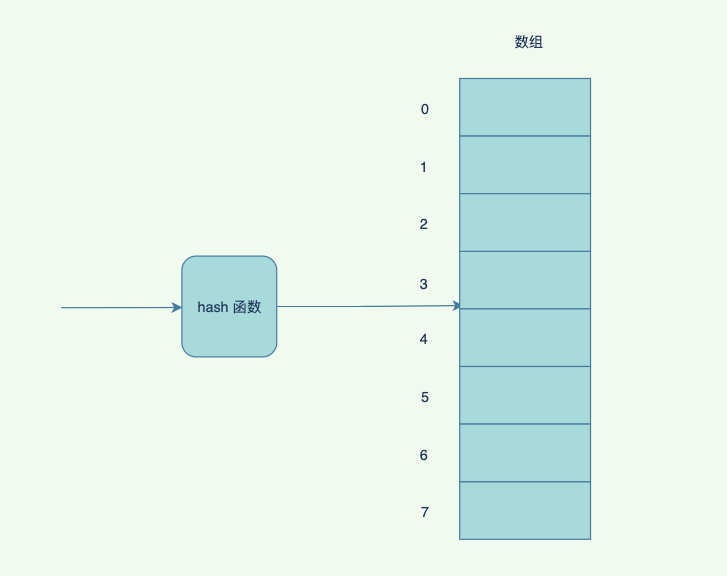• 直接定址法：取出關鍵字或者關鍵字的某個線性函式的值為雜湊函式，比如`H(key) = key`或者`H(key) = a * key + b`
• 數字分析法：對於可能出現的數值全部瞭解，取關鍵字的若干數位組成雜湊地址
• 平方取中法：取關鍵字平方後的中間幾位作為雜湊地址
• 摺疊法：將關鍵字分割成為位數相同的幾部分（最後一部分的位數可以不同），取這幾部分的疊加和（捨去進位），作為雜湊地址。
• 除留餘數法：取關鍵字被某個不大於散列表表長`m`的數`p`除後所得的餘數為雜湊地址。即h`ash(k)=k mod p``p< =m`。不僅可以對關鍵字直接取模，也可在摺疊法、平方取中法等運算之後取模。對`p`的選擇很重要，一般取素數或`m`，若`p`選擇不好，容易產生衝突。
• 隨機數法：取關鍵字的隨機函式值作為它的雜湊地址。

• 開放地址法：`hash`計算後，如果該位置已經有資料，那麼對該地址`+1`，也就是往後找，知道找到一個空的位置。
• 重新`hash`法：發生雜湊衝突後，可以使用另外的`hash`函式重新極計算，找到空的`hash`地址,如果有，還可以再疊加`hash`函式。
• 鏈地址法：所有`hash`值一樣的,連結成為一個連結串列，掛在陣列後面。
• 建立公共溢位區：不常見，意思是所有元素，如果和表中的元素`hash`衝突，都弄到另外一個表，也叫溢位表。

`Java`裡面，用的就是鏈地址法：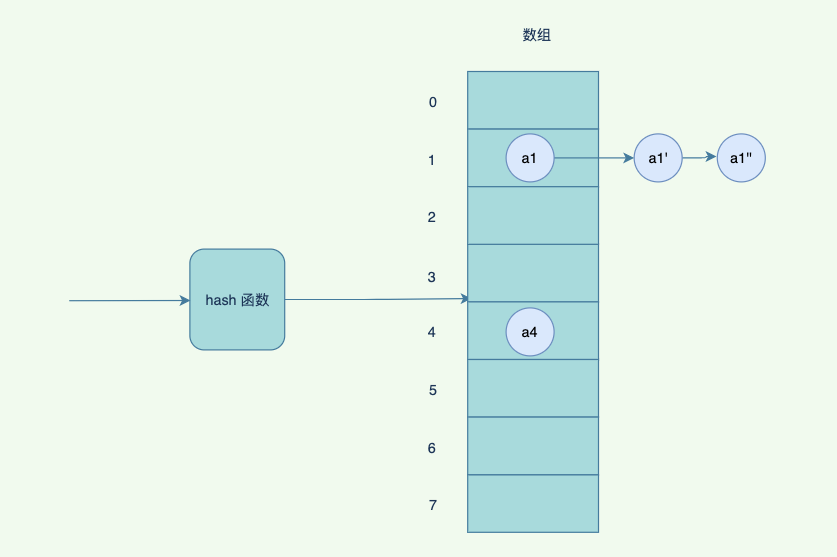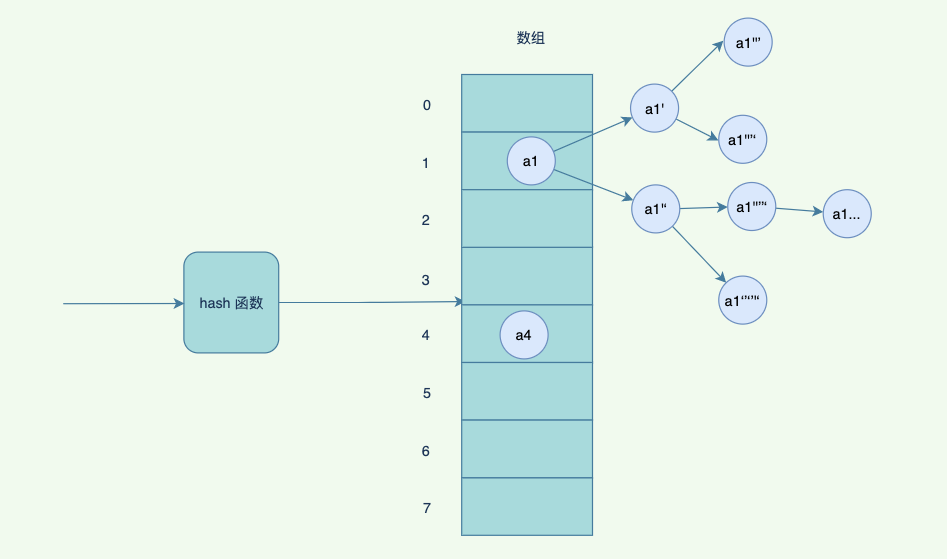`hash`表的優點是查詢速度快，但是如果不斷觸發重新 `hash`, 響應速度也會變慢。同時，如果希望範圍查詢，`hash`表不是好的選擇。

## 樹• 節點的度：一個節點含有的子樹的個數稱為該節點的度
• 樹的度：一棵樹中，最大的節點度稱為樹的度；
• 葉節點或終端節點：度為零的節點；
• 非終端節點或分支節點：度不為零的節點；
• 父親節點或父節點：若一個節點含有子節點，則這個節點稱為其子節點的父節點；
• 孩子節點或子節點：一個節點含有的子樹的根節點稱為該節點的子節點；
• 兄弟節點：具有相同父節點的節點互稱為兄弟節點；
• 節點的層次：從根開始定義起，根為第`1`層，根的子節點為第`2`層，以此類推；
• 深度：對於任意節點`n`,`n`的深度為從根到n的唯一路徑長，根的深度為`0`
• 高度：對於任意節點`n`,`n`的高度為從`n`到一片樹葉的最長路徑長，所有樹葉的高度為`0`
• 堂兄弟節點：父節點在同一層的節點互為堂兄弟；
• 節點的祖先：從根到該節點所經分支上的所有節點；
• 子孫：以某節點為根的子樹中任一節點都稱為該節點的子孫。
• 有序樹：將樹種的節點的各個子樹看成從左至右是有次序的（不能互換），則應該稱該樹為有序樹，否則為無序樹
• 第一個孩子：在有序樹中最左邊的子樹的根稱為第一個孩子
• 最後一個孩子：在有序樹種最右邊的子樹的根稱為最後一個孩子
• 森林：由`m``m>=0`）棵互不相交的樹的集合稱為森林；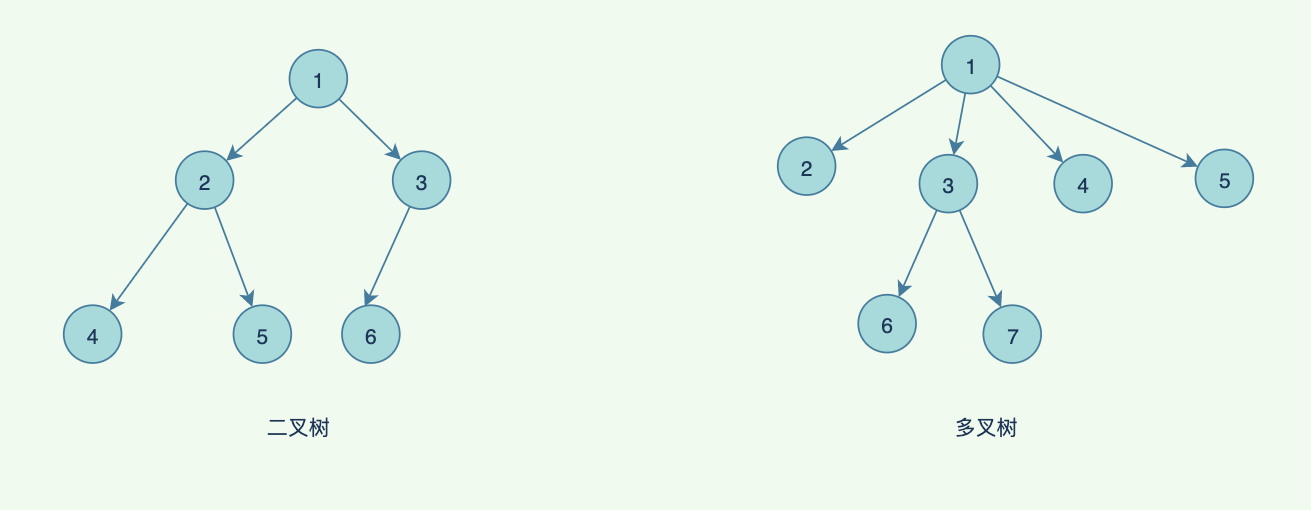``````public class TreeLinkNode {
int val;

this.val = val;
}
}
``````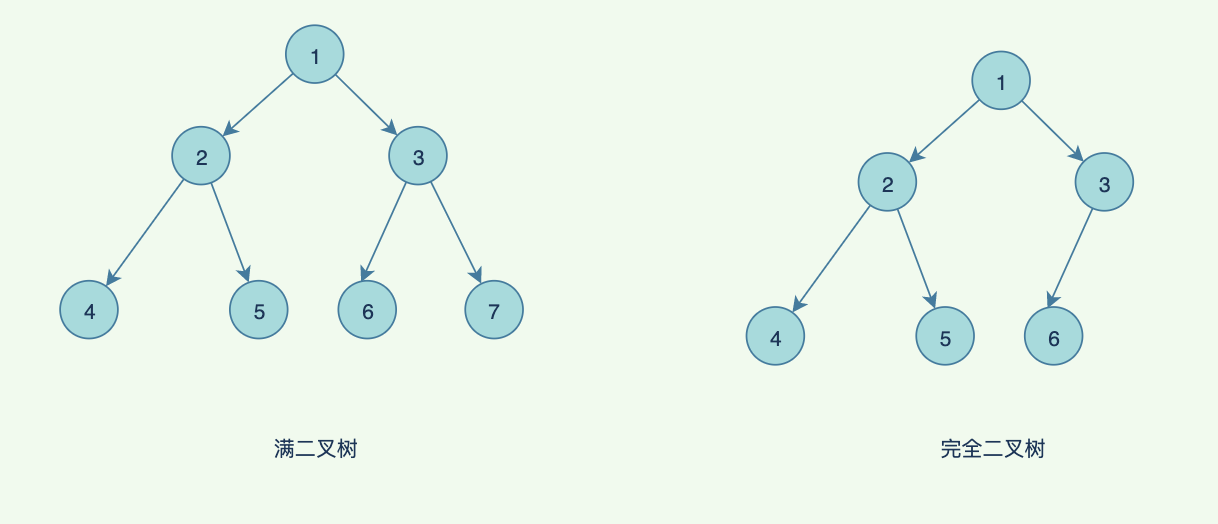• 前序遍歷：遍歷順序 根節點 --> 左子節點 --> 右子節點
• 中序遍歷：遍歷順序 左子節點 --> 根節點 --> 右子節點
• 後序遍歷：遍歷順序 左子節點 --> 右子節點 --> 根節點
• 廣度 / 層次遍歷： 從上往下，一層一層的遍歷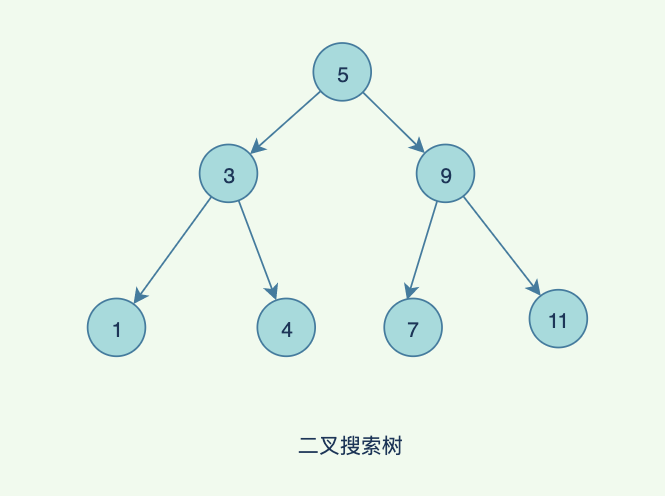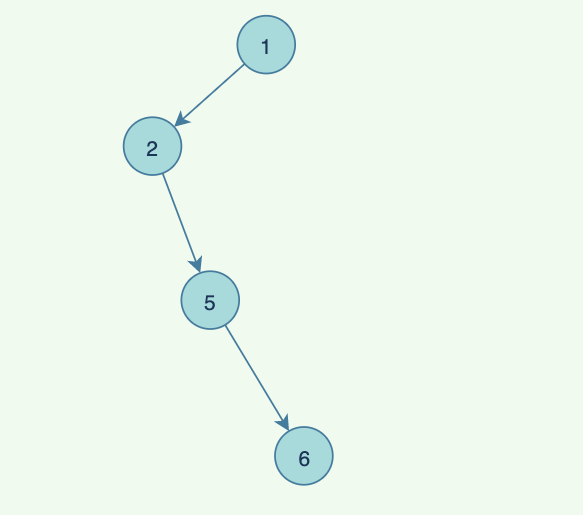• 性質1. 結點是紅色或黑色。

• 性質2. 根結點是黑色。

• 性質3. 所有葉子都是黑色。（葉子是NIL結點）

• 性質4. 每個紅色結點的兩個子結點都是黑色。（從每個葉子到根的所有路徑上不能有兩個連續的紅色結點）

• 性質5. 從任一節結點其每個葉子的所有路徑都包含相同數目的黑色結點。

970年，R.Bayer和E.mccreight提出了一種適用於外查詢的，它是一種平衡的多叉樹，稱為B樹（或B-樹、B_樹）。

1、根結點至少有兩個子女；

2、每個非根節點所包含的關鍵字個數 j 滿足：m/2 - 1 <= j <= m - 1；

3、除根結點以外的所有結點（不包括葉子結點）的度數正好是關鍵字總數加1，故內部子樹個數 k 滿足：m/2 <= k <= m ；

4、所有的葉子結點都位於同一層。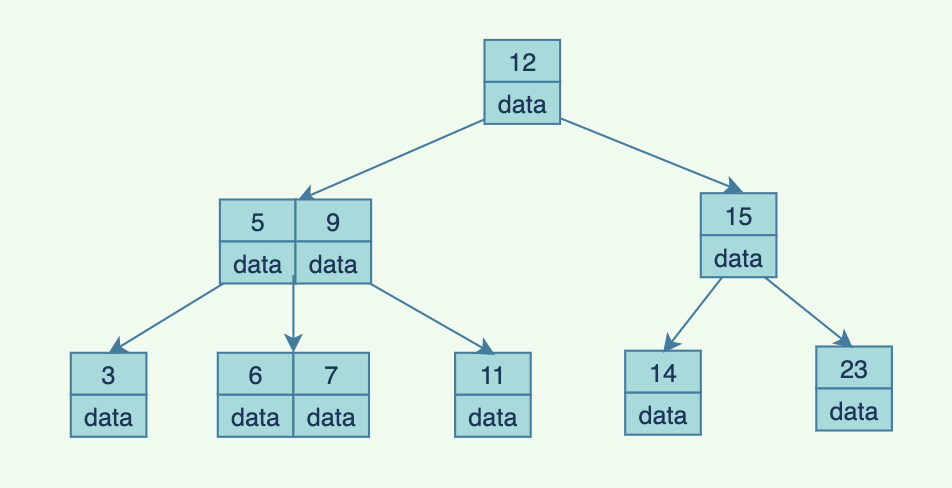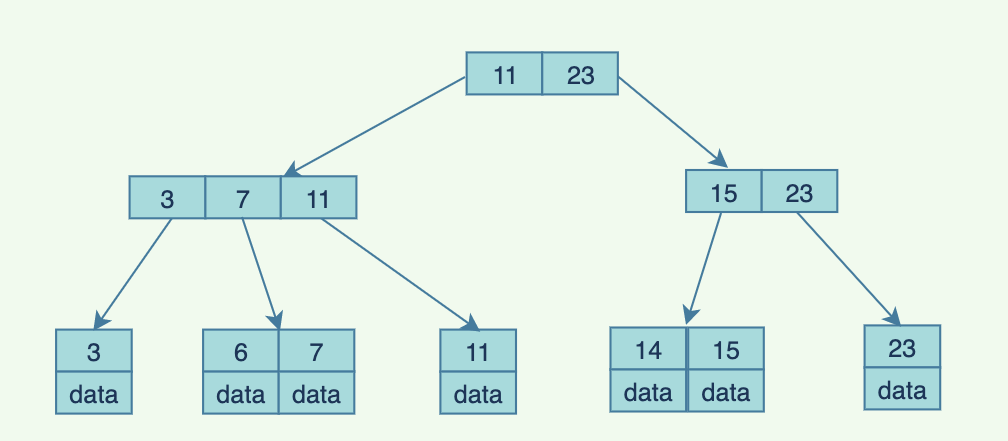B+樹是B樹的一種變形形式，B+樹上的葉子結點儲存關鍵字以及相應記錄的地址，葉子結點以上各層作為索引使用。一棵m階的B+樹定義如下:

(1)每個結點至多有m個子女；

(2)除根結點外，每個結點至少有[m/2]個子女，根結點至少有兩個子女；

(3)有k個子女的結點必有k個關鍵字。

1. `b+`樹的中間節點不儲存資料，每次IO查詢能查到更多的索引，,是一個矮胖的樹。
2. 對於範圍查詢來說，`b+`樹只需遍歷葉子節點連結串列即可，`b`樹卻需要從根節點都葉子節點。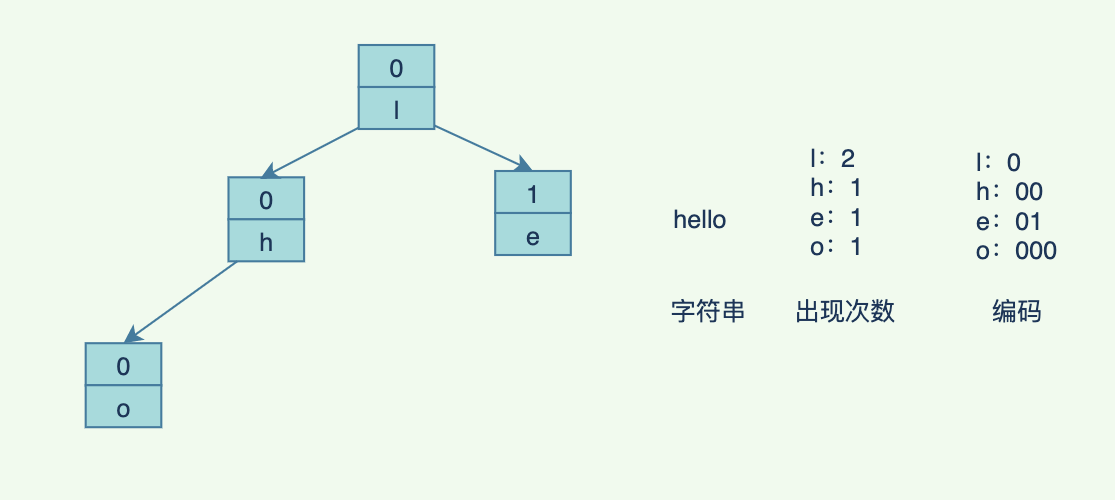## 堆

• 大頂堆：每個節點都大於等於其子樹節點（堆頂是最大值）
• 小頂堆：每個節點都小於等於其子樹節點（堆頂是最小值）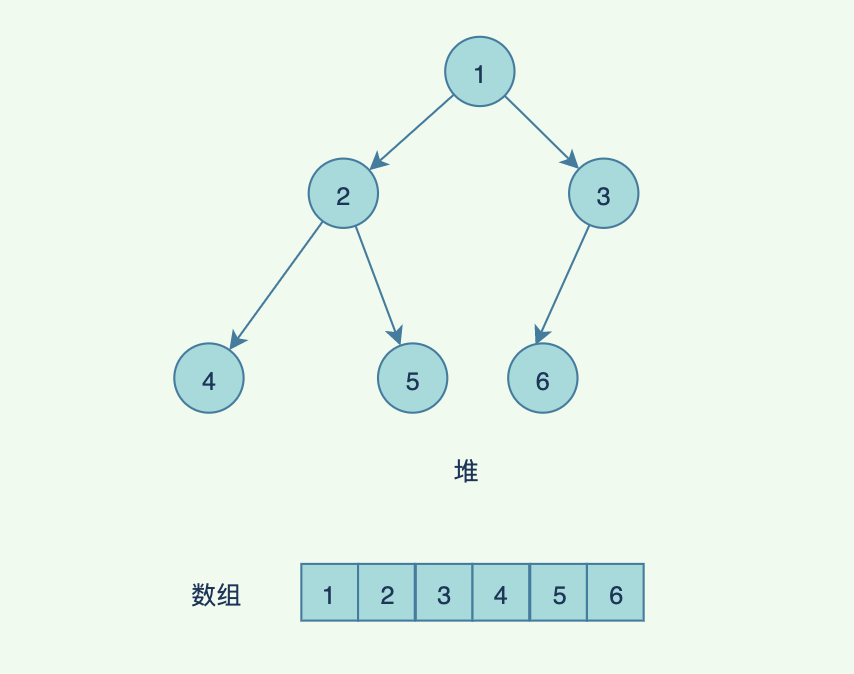• `i `結點的父結點 `parent = floor((i-1)/2) `(向下取整)
• `i `結點的左子結點 `2 * i +1`
• `i `結點的右子結點 `2 * i + 2`

• 還是完全二叉樹
• 堆中每一個節點都還小於等於其左右子節點

## 圖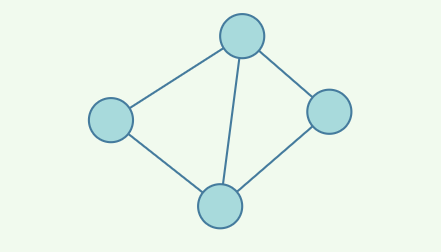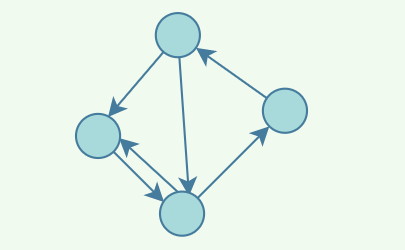• 頂點：圖最基本的單元，那些節點
• 邊：頂點之間的關聯關係
• 相鄰頂點：由邊直接關聯的頂點
• 度：一個頂點直接連線的相鄰頂點的數量
• 權重：邊的權值

1. 鄰接矩陣，使用二維陣列表示，為1 表示聯通，0表示不連通，當然如果表示路徑長度的時候，可以用大於`0`的數表示路徑長度，用`-1`表示不連通。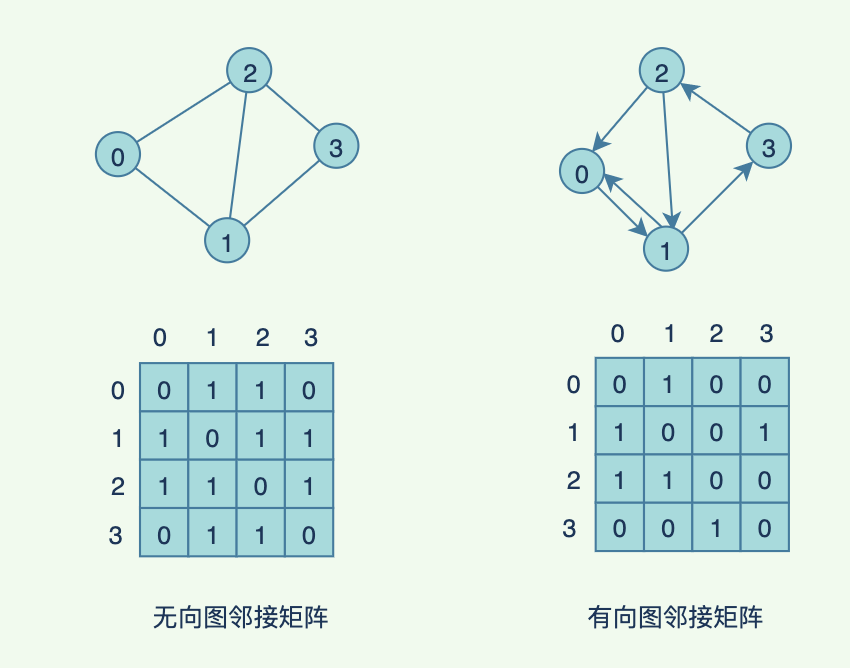1. 鄰接表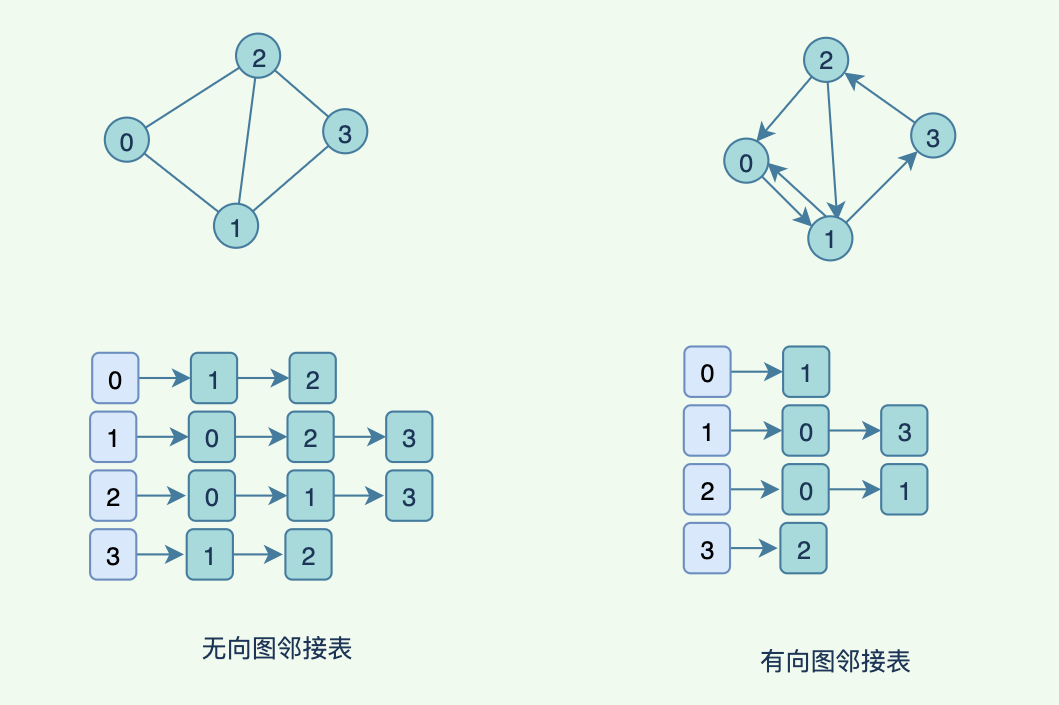## 秦懷@觀點【作者簡介】